Courses

# Problems on Playing Cards - Probability, CBSE, Class 10, Mathematics Class 10 Notes | EduRev

## Class 10 : Problems on Playing Cards - Probability, CBSE, Class 10, Mathematics Class 10 Notes | EduRev

The document Problems on Playing Cards - Probability, CBSE, Class 10, Mathematics Class 10 Notes | EduRev is a part of Class 10 category.
All you need of Class 10 at this link: Class 10

DESIGNATION OF PLAYING CARDS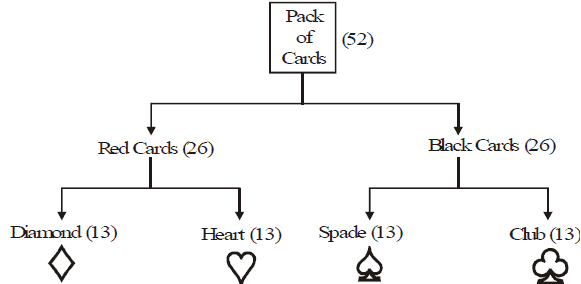(i) A deck (pack) of cards contains 52 cards, out of which there are 26 red cards and 26 black cards.

(ii) There are four suits each containing 13 cards.

(iii) The cards in each suit are ace (A), king, queen, jack, 10, 9, 8, 7, 6, 5, 4, 3 and 2.

(iv) Kings, queens and jacks are called face cards (4 + 4 + 4 = 12) .

(v) Kings, queens, jacks and aces are called honour cards (4 + 4 + 4 + 4 = 16).

JUST FOR YOU
1. P(E) =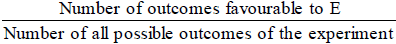2. Probability of an impossible event = 0.

3. Probability of a sure event = 1.

4. P(E) + P(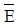) = 1, where E and E are complementary events.

5. 0 < P(E) < 1

6. The sum of the probabilities of all the elementary events of an experiment is 1.

Ex.1 Complete the following statements :

(i) Probability of an event E + probability of the event 'not E' = ..........

(ii) The probability of an event that is certain to happen is.......... Such an event is called..........

(iii) The probability of an event is greater than or equal to.......and less than or equal to......

(iv) P(E) = ............

Total number of trials

Sol. (i) 1

(ii) 1, sure or certain event

(iii) 0, 1

(iv) number of trials in which event
happened.

Ex.2  Which of the following experiments have equally likely outcomes? Explain.

(i) A driver attempts to starts a car. The car starts or does not start.

(ii) A player attempts to shoot a basketball. She/he shoots or misses the shot.

(iii) A coin is tossed. It turns to be a head or a tail.

(iv) A monitor is nominated by the class teacher of a class. It is a boy or a girl.

Sol. (iii) because when a coin is tossed either a head or a tail turns.

Ex.3 Match the following:

(i) P() =                                                                         (a) 0

(ii) Probability of an impossible event                              (b) 0.5

(iii) Probability of an event cannot be more than              (c) 1 – P(E)

(iv) A card is drawn from a pack of 52 cards, then the

probability of getting a red card is equal to                      (d) 1

Sol. (i) (c), (ii) (a), (iii) (d), (iv) (b)

Ex.4  If P(E) = 0.05, what is the probability of 'not E'?
Sol. We have P(E) = 0.05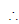P(not E) = 1 – P(E) = 1 – 0.05 = 0.95
Therefore, P(not E) = 0.95.

Ex.5 Find the probability of getting a head when a coin is tossed once?

Sol. When a coin is tossed, then all possible outcomes are H and T
Total number of possible outcomes = 2
Let E be the event of getting a headnumber of favourable outcome = 1
Hence, required probability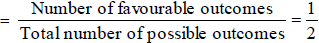Ex.6 Two unbiased coins are tossed simultaneously. Find the probability of getting
(i) one head

(ii) one tail

(iii) two heads

(iv) at least one head

(v) at most one head

(vi) no head.

Sol. If two unbiased coins are tossed simultaneously, then all possible outcomes are:
HH, HT, TH, TT.
Total number of possible outcomes = 4.

(i) Let A1 = the event of getting one head.
Then, favourable outcomes are HT, TH .
Number of favourable outcomes = 2 .
Hence, required probability = P (getting one head)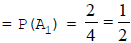(ii) Let A2 = the event of getting one tail.
Then, favourable outcomes are TH, HT.
Number of favourable outcomes = 2 .
Hence, required probability = P (getting one tail)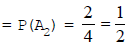(iii) Let A3 = the event of getting two heads
Then, the favourable outcome is HH
Number of favourable outcome = 1
Hence, required probability = P (getting two heads)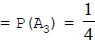(iv) Let A4 = the event of getting at least one head. 4
Then, the favourable outcomes are HT, TH, HH
Number of favourable outcomes = 3
Hence, required probability = P (getting at least one head)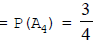(v) Let A5 = the event of getting atmost one head.
Then, the favourable outcomes are TT, HT, TH.
Number of favourable outcomes = 3
Hence, required probability = P (getting atmost one head)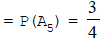(vi) Let A6 = the event of getting no head.
Then, the favourable outcomes is TT
Number of favourable outcome = 1
Hence, required probability = P (getting one head)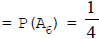Ex.7 Three unbiased coins are tossed together. Find the probability of getting
(i) one head

(ii) two heads

(iii) all heads

(iv) at least two heads

Sol. If three unbiased coins are tossed together, then all possible outcomes are :
HHH, HHT, HTH, THH, HTT, THT, TTH, TTT
Total number of possible outcomes = 8
(i) Let A1 = the event of getting one head.
Then, the favourable outcomes are HTT, THT, TTH
Number of favourable outcomes = 3
Hence, required probability = P (getting one head)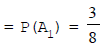(ii) Let A2 = the event of getting two heads.
Then, the favourable outcomes are HHT, HTH, THH.
Number of favourable outcomes = 3
Hence, required probability = P (getting two heads)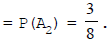.
(iii) Let A3 = event of getting all heads.
Then, the favourable outcomes is HHH
Number of favourable outcome = 1
Hence, required probability = P (getting all heads)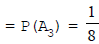(iv) Let A4 = event of getting at least two heads.
Then, the favourable outcomes are HHT, HTH, THH, HHH
Number of favourable outcomes = 4
Hence, required probability = P (getting at least two heads)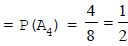Ex.8 A die is thrown once. Find the probability of getting

(i) a prime number

(ii) a number lying between 2 and 6

(iii) an odd number.

Sol. If a die is thrown, then all possible outcomes are 1, 2, 3, 4, 5, 6.
Total number of possible outcomes = 6 .

(i) Let A1 = event of getting a prime number.
Then, the favourable outcomes are 2, 3, 5.
Number of favourable outcomes = 3
Hence, required probability = P (getting a prime number)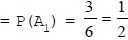(ii) Let A2 = event of getting a number lying between 2 and 6.
Then, the favourable outcomes are 3, 4, 5.
Number of favourable outcomes = 3 .

Hence, required probability = P (getting a number lying between 2 and 6)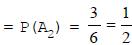(iii) Let A3 = event of getting an odd number.
Then, the favourable outcomes are 1, 3, 5.
Number of favourable outcomes = 3 .
Hence, required probability = P (getting an odd number)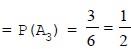Ex.9 A die is thrown twice. What is the probability that

(i) 5 will not come up either time?

(ii) 5 will come up at least once?

Sol. If a die is thrown twice, then all the possible outcomes are:
(1,1 ),(1,2),(1,3),(1,4),(1,5),(1,6),
(2,1), (2,2), (2,3), (2,4), (2,5), (2,6),
(3,1), (3,2), (3,3), (3,4), (3,5), (3,6),
(4,1 ),(4,2),(4,3 ),(4,4),(4,5 ),(4,6),
(5,1 ),(5,2),(5,3 ),(5,4),(5,5),(5,6),
(6,1 ),( 6,2),( 6,3 ),(6,4),( 6,5),( 6,6).

Total number of outcomes = 36 .

(i) Let A1 = event of getting 5 not either time.

Then, the favourable outcomes are: (1,1),(1,2),(1,3),(1,4),(1,6),
(2,1),(2,2),(2,3),(2,4),(2,6),
(3,1),(3,2),(3,3),(3,4),(3,6),
(4,1),(4,2),(4,3),(4,4),(4,6),
(6,1),(6,2),(6,3),(6,4),(6,6).
Number of favourable outcomes = 25

Hence, required probability = P (5 will not come up either time)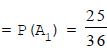(ii) Let A2 = event of getting 5 at least once.
Then, the favourable outcomes are
(1,5),(2,5),(3,5),(4,5),(5,1),(5,2),(5,3),(5,4),(5,5),(5,6),(6,5).
Number-of favourable outcomes = 11
Hence, required probability = P (5 will come up at least once)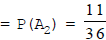Ex.10 A pair of dice is thrown simultaneously. Find the probability of getting
(i) a doublet
(ii) sum of the numbers on two dice is always 7
(iii) an even number on the first die and a multiple of 3 on the other.

Sol. If a pair of dice is thrown simultaneously, then all the possible outcomes are:
(1,1),(1,2),(1,3),(1,4),(1,5), (1,6),(2,1),(2,2),(2,3),(2,4),(2,5),(2,6),(3,1),(3,2),(3,3),(3,4),(3,5),(3,6), (4,1),(4,2),
(4,3),(4,4),(4,5),(4,6),(5,1),(5,2),(5,3),(5,4),(5,5),(5,6),(6,1),(6,2),(6,3),(6,4),(6,5),(6,6).
Total number of possible outcomes = 36

(i) Let A1 = event of getting a doublet.
Then, the favourable outcomes are (1,1),(2,2),(3,3),(4,4),(5,5),(6,6).
Number of favourable outcomes = 6
Here, required probability = P (getting a doublet)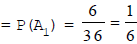(ii) Let A2 = event of getting a sum of the numbers on two dice is always 7.
Then, the favourable outcomes are (1,6),(2,5),(3,4),(4,3),(5,2),(6,1)
Number of favourable outcomes = 6
Here, required probability = P (getting a sum of the numbers on two dice is always 7)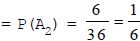(iii) Let A3 = event of getting an even number on the first die and a multiple of 3 on the other.
Then, the favourable outcomes are (2,3),(2,6),(4,3),(4,6),(6,3),(6,6).
Number of favourable outcomes = 6
Here, required probability = P (getting an even number on the first die and a multiple of 3 on the other)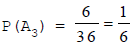Offer running on EduRev: Apply code STAYHOME200 to get INR 200 off on our premium plan EduRev Infinity!

,

,

,

,

,

,

,

,

,

,

,

,

,

,

,

,

,

,

,

,

,

,

,

,

,

,

,

,

,

,

;